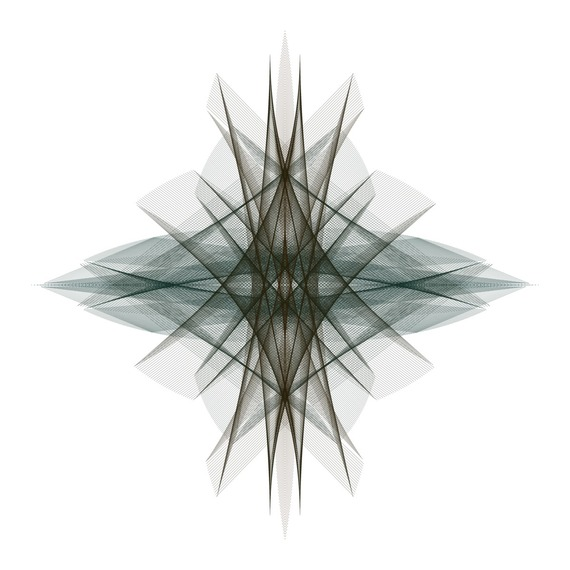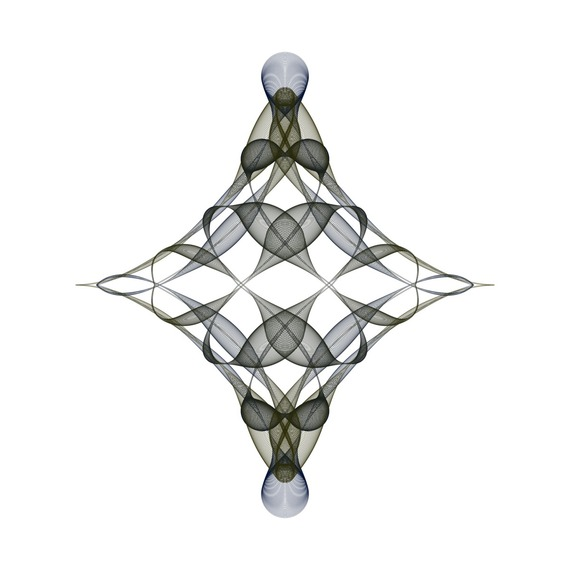# Using the Sawtooth Wave to Create Some Beautiful Images

When we want to create a beautiful image by drawing line segments or circles, we can use sawtooth waves. The sawtooth wave is a useful waveform to introduce an unlimited number of symmetrical shapes. Let S(x)=2x-2[x]-1, where [x] is the largest integer less than or equal to x. S(x) is a sawtooth wave. This periodic function is not continuous at the integers. I have created the following images by using S(x) in their formulas. At the end of this post you can see the mathematical descriptions of "3,000 Line Segments (III)" and "7000 Circles (II)".

3,000 Line Segments (III)This image shows 3,000 line segments. For each k=1,2,3,...,3000 the endpoints of the k-th line segment are:

(S(20k/3000)cos(2πk/3000), S((18k/3000)+(1/2))(sin(2πk/3000))^3)

and

(S(16k/3000)(cos(2πk/3000))^3, S((14k/3000)+(1/2))sin(2πk/3000)).

7,000 Circles (II)This image shows 7,000 circles. For each k=1,2,3,...,7000 the center of the k-th circle is:

(S((14k/7000)+(1/2))sin(10πk/7000), S(14k/7000)cos(10πk/7000))

and the radius of the k-th circle is:

(1/400)+(1/9)(S(14k/7000)cos(50πk/7000))^2.